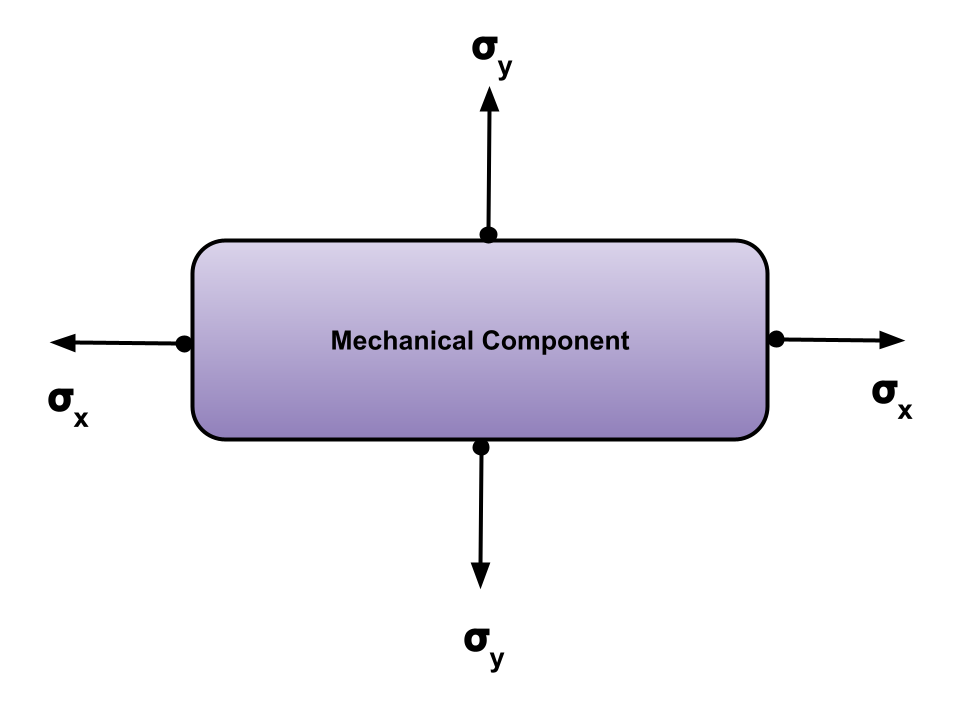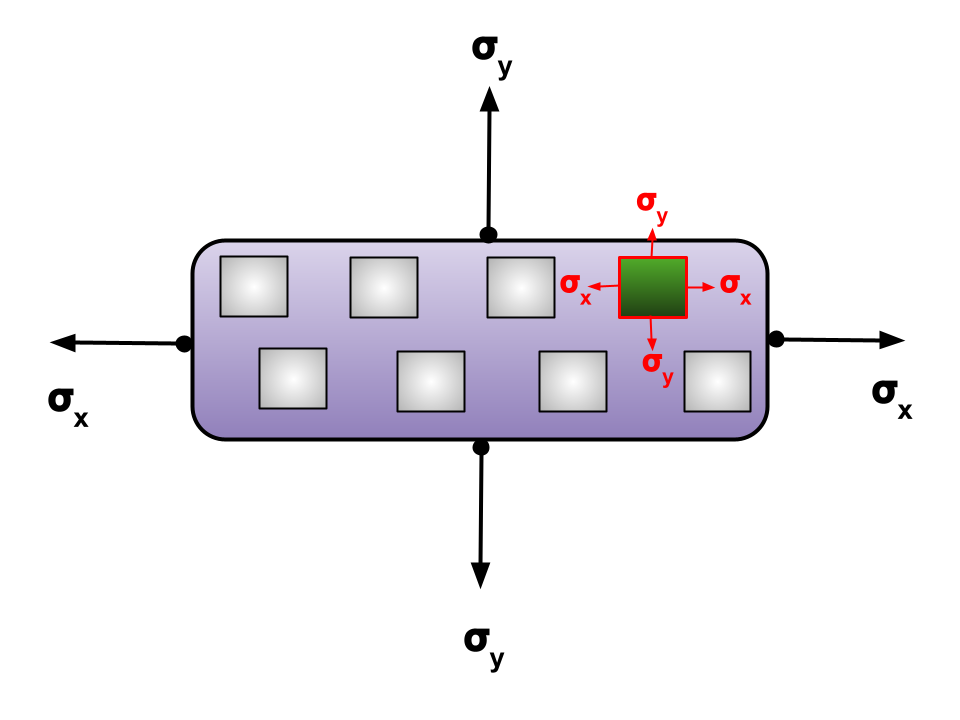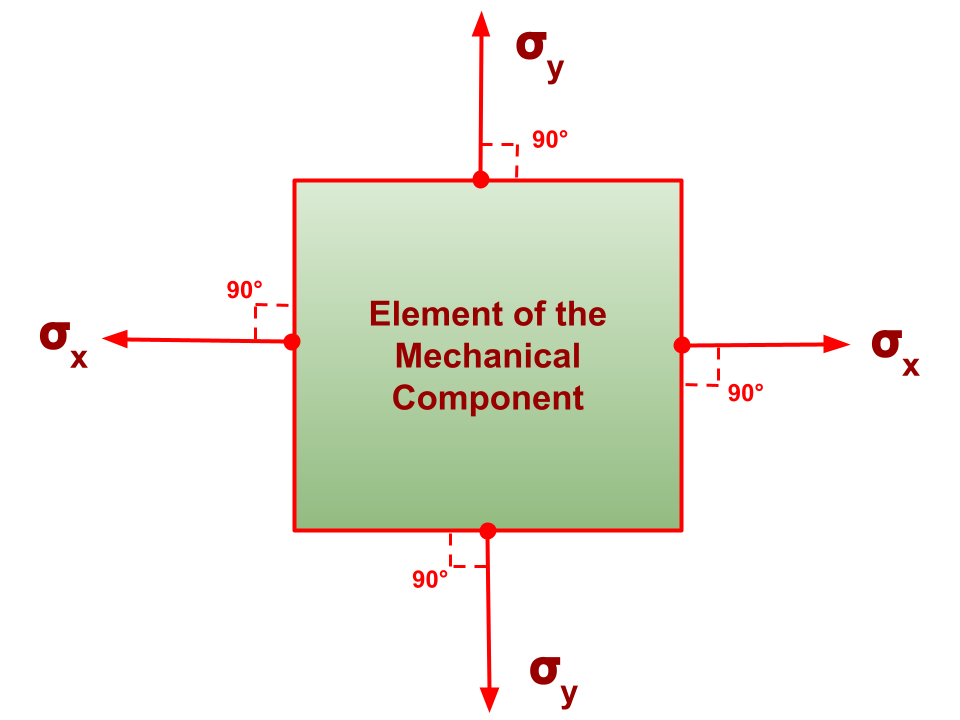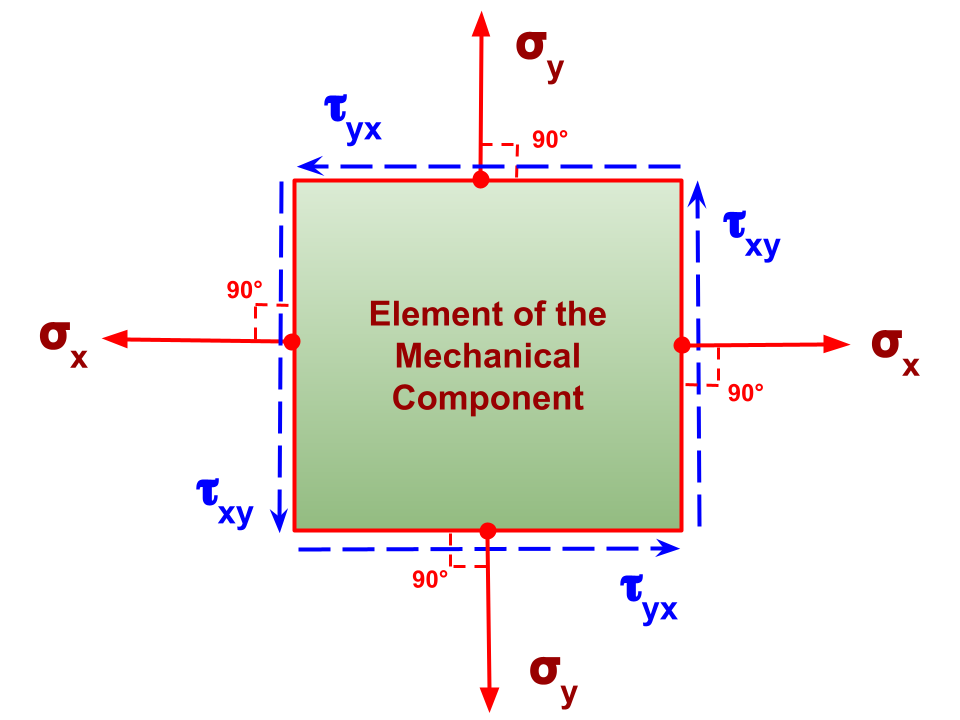# Principal Stress Calculator

By Juhi Raj, PhD candidate
Last updated: Oct 15, 2021

This principal stress calculator will help you discover and learn about maximum and minimum principal stress and the principal plane. With our website, you can quickly input the stress components and get your results in no time! The principal stress formulas will help you compute the extremes of typical stress that a plane can experience at any given time - in other words, we'll find the amplitudes of normal stress operating on a primary plane. And if you want to better grasp what the principal stress is, wait no longer and keep reading!

You can also solve other problems in mechanics involving stress, strain, and Young's modulus using our stress calculator. If you would like to compute how to draw Mohr's circle, use our Mohr's circle tool.

## What is principal stress?

Principal stress is the normal stress a body can have at some point. In the instances where more than two stresses are present (i.e. compound stress), the resultant stress at a point consists of both the normal and shear stress. In both cases of stress at that point, the stress is the function of the inclination to the horizontal. Or in other words, principal stress is the minimum and maximum stresses derived from normal stress at an angle on a plane where shear stress is zero.

Let us take a look at an exemplary mechanical component, as shown below:• The mechanical component is subjected to biaxial stress conditions, `σx` and `σy`.

• Consider small elements inside this mechanical component, as shown below:• The grey boxes inside the mechanical component represent the exemplary small elements inside the mechanical component.
• If you focus on the stress of each of the elements, you will find that the stress is the same, `σx` in the horizontal direction and `σy` in the vertical direction on every single element of the component.
• One such element is colored green and emphasized to aid in your understanding.

Before we move ahead, let us define the principal plane. A principal plane is a plane on which only normal stresses will act. In other words, there is no shear stress acting on the plane.

Let us take a look at one of the small elements with unit thickness from the mechanical component as shown in the figure below:• The stresses in the horizontal (`σx`) and vertical (`σy`) directions are acting normally (at 90°) to the principal plane, therefore, they both are called normal stresses.

• Take a look at the same element, which is part of the mechanical component, but this time with additionally applied shear stresses, i.e., `τxy` acting along the horizontal direction to the vertical direction and `τyx` acting along the vertical direction to the horizontal direction.

• The shear stresses are represented with the blue dashed arrows along the normal plane, as shown below:The applied shear is rotating the body in an anti-clockwise direction. The principal plane will lie with an inclination at an angle `α` from the vertical plane inside the surface. Now, the question arises as to what is principal stress? The normal stresses acting on the principal plane are called the principal stresses.

## What is the principal stress calculation used for?

If any material is brittle in nature, it is going to fail due to the principal stresses and not due to the shear stresses. Also, the maximum principal stress is responsible for any deterioration of the material. Therefore, components are designed to withstand the maximum principal stress. The fracture of the material will take place along the principal plane.

## How to use our principal stress calculator?

Follow the steps below to use this principal stress calculator with some exemplary values:

1. Enter the normal stress in the horizontal direction, `σ_x`.

2. Input the normal stress in the vertical direction, `σ_y`.

3. Plugin the shear stress, `τ_xy`.

4. The shear stress, `τ_yx`, has the same amplitude as `τ_xy`, but it acts in the opposite direction.

The principal stress calculator will now use the principal stress equations to calculate maximum and minimum principal stresses and the angle of principal stress. The angle of principal stress is commonly computed in radians. You can also use our angle converter to get the result in degrees - or simply convert it directly in this principal stress calculator.

Let us take a look at an example of how to use this tool.

Example: If the horizontal stress is 10 MPa, vertical stress is 10 MPa, and shear stress is 8 MPa, we can compute the principal stresses as follows:

1. Input horizontal stress ` (σ_x) = 10 MPa`.

2. Input vertical stress ` (σ_y) = 10 MPa`.

3. Enter the shear stress ` (σ_xy) = 8 MPa`.

The calculator will show you results as follows:

• The maximum principal stress ` (σ_max) = 18 MPa `;

• The minimum principal stress` (σ_min) = 2 MPa `;

• Angle of principal stress ` (θ) = 45° `;

• Angle of maximum shear stress ` (θ_τmax) = 90° `; and

• Angle of minimum shear stress ` (θ_τmin) = 0° `.

## Principal stress formula

If you would like to calculate the principal stresses on your own, you can use the principal stress equations presented below:

1. The maximum principal stress equation is:

`σmax = ((σx+ σy)/2) + √(((σx - σy)/2)2+ (τxy2))`

1. The minimum principal stress equation is:

`σmin = ((σx+ σy)/2) - √(((σx - σy)/2)2+ (τxy2))`

1. The angle of principal stress is calculated as shown below:

`θ = (tan-1((2 * τxy) / (σx - σy)))/2`

The orientations of maximum shear stress occur at 45° to the principal plane.

1. The maximum angle of shear stress is computed as:

`θτmax = (tan-1((2 * τxy) / (σx - σy)))/2 + tan-1(1) `

1. The minimum angle of shear stress is computed as:

`θτmax = (tan-1((2 * τxy) / (σx - σy)))/2 - tan-1(1) `

Juhi Raj, PhD candidate
Normal Stresses
Horizontal normal stress (σ_x)
lbs/ft²
Vertical normal stress (σ_y)
lbs/ft²
Shear Stresses
XY shear stress (τ_xy)
lbs/ft²
YX shear stress (τ_yx)
lbs/ft²
Principal Stresses
Maximum principal stress (σ_max)
lbs/ft²
Minimum principal stress (σ_min)
lbs/ft²
Orientation of the Stress Components
Angle of principal stress (θ)
Angle of maximum shear stress (θ_τmax)
Angle of minimum shear stress (θ_τmin)
People also viewed…

### BMR - Harris-Benedict equation

Harris-Benedict calculator uses one of the three most popular BMR formulas. Knowing your BMR (basal metabolic weight), may help you make important decisions about your diet and lifestyle.

### Brewster Angle

With this Brewster's Angle Calculator, you can estimate the angle at which reflected light will be perfectly polarized.

### Normal force

Find out the normal force value of an object on a flat and inclined surface with our normal force calculator.